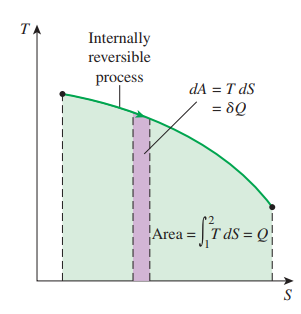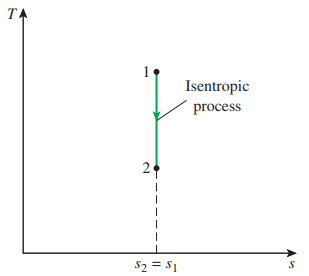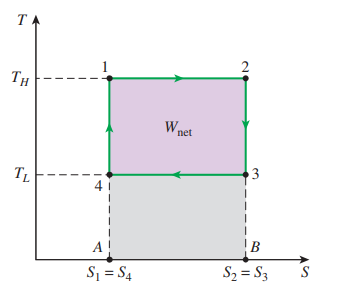Need Help?

Subscribe to Thermodynamics

###### \${selected_topic_name}
• Notes
• Comments & Questions
• The entropy change of a specified mass m (a closed system) during a process is simply

$\Delta S=m \Delta s=m\left(s_{2}-s_{1}\right) \quad(\mathrm{kJ} / \mathrm{K})$

Which is the difference between the entropy values at the final and initial states.

• The entropy ofa fixed mass does not change during a process that is internally reversibleand adiabatic. This process is called isentropic process

$\Delta s=0 \quad$ or $\quad s_{2}=s_{1} \quad(\mathrm{k} \mathrm{J} / \mathrm{kg} \cdot \mathrm{K})$

• Property diagrams involving entropy

T-S diagramT-s diagram for carnot cycleNo comments yet